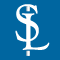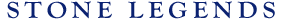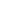HOMEPRODUCTSIDEA CENTERSERVICESTECHNICALABOUT USHello Guest Login  |  Become Member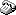800-398-1199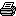214-398-1293MapEmail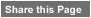Click to Share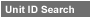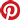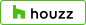Select which variables you would like to search by.Type DescriptionPrimary ViewPrimary ModifierUnit NameView Definitions

View Definitions

View DefinitionsX-Dim Y-Dim Z-Dim XX-Dim YY-Dim ZZ-Dim R-Dim D-Dim P-Dim
 Click column heading to re-sort results list.Product Name Primary View Modifier Pri Dimx  y  z Alt Dimxx  yy  zz Alt2 Dimr  d  p E\$timated Thumbnail Chamfer1 AddressBlock Rectangular X = 8-1/2" Y = 12"Z = 4" XX = 7" YY = n/aZZ = 3-1/4" R = n/a D = n/aP = 3-1/4" \$ 105.00Chamfer2 AddressBlock Rectangular X = 9" Y = 14"Z = 4-3/4" XX = n/a YY = n/aZZ = n/a R = n/a D = n/aP = n/a \$ 120.00Chamfer3 AddressBlock Square X = 7-1/2" Y = 7-1/2"Z = 4" XX = 6-1/2" YY = 6-1/2"ZZ = 3-1/2" R = n/a D = n/aP = n/a \$ 88.35Chamfer4 AddressBlock Rectangular X = 18" Y = 36"Z = 4" XX = 16" YY = n/aZZ = 3" R = n/a D = n/aP = 3" \$ 408.00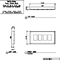Chamfer5 AddressBlock Rectangular X = 17" Y = 32"Z = 3-1/2" XX = n/a YY = n/aZZ = 2-3/4" R = n/a D = n/aP = 2-3/4" \$ 257.86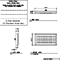Extrados1 AddressBlock Rectangular X = 11" Y = 18-3/4"Z = 4" XX = n/a YY = n/aZZ = n/a R = n/a D = n/aP = n/a \$ 180.00Medallion1 AddressBlock Rectangular X = 11-1/2" Y = 18-1/2"Z = 4" XX = n/a YY = n/aZZ = n/a R = n/a D = n/aP = n/a \$ 150.00Medallion2 AddressBlock Rectangular X = 11-1/2" Y = 17"Z = 4" XX = n/a YY = n/aZZ = n/a R = n/a D = n/aP = n/a \$ 150.00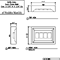Medallion3 AddressBlock Rectangular X = 8-1/2" Y = 15"Z = 4" XX = n/a YY = n/aZZ = n/a R = n/a D = n/aP = n/a \$ 135.00Medallion4 AddressBlock Rectangular X = 18" Y = 24"Z = 4" XX = n/a YY = n/aZZ = n/a R = n/a D = n/aP = n/a \$ 207.90Merit1 AddressBlock Square X = 12" Y = 12"Z = 4" XX = 8" YY = 8"ZZ = n/a R = n/a D = n/aP = n/a \$ 135.00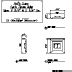Recess1 AddressBlock Rectangular X = 8-1/2" Y = 12"Z = 3-1/2" XX = n/a YY = n/aZZ = n/a R = n/a D = n/aP = n/a \$ 105.00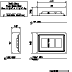Recess2 AddressBlock Rectangular X = 8-1/2" Y = 15"Z = 4" XX = n/a YY = n/aZZ = n/a R = n/a D = n/aP = n/a \$ 135.00Recess3 AddressBlock Rectangular X = 8-1/2" Y = 26-1/4"Z = 4" XX = n/a YY = n/aZZ = n/a R = n/a D = n/aP = n/a \$ 143.66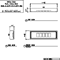Recess4 AddressBlock Rectangular X = 8-1/2" Y = 16"Z = 4" XX = 7" YY = 14"ZZ = 3-1/4" R = n/a D = n/aP = n/a \$ 137.00Reflection1 AddressBlock Rectangular X = 11" Y = 16"Z = 5" XX = n/a YY = 12-1/2"ZZ = n/a R = n/a D = n/aP = n/a \$ 190.00Reprise1 AddressBlock Rectangular X = 11-1/8" Y = 16-3/4"Z = 4" XX = n/a YY = n/aZZ = n/a R = n/a D = n/aP = n/a \$ 180.00Reprise2 AddressBlock Rectangular X = 14" Y = 20"Z = 4" XX = n/a YY = n/aZZ = n/a R = n/a D = n/aP = n/a \$ 236.00Tracery1 AddressBlock Rectangular Art X = 11-1/8" Y = 18-1/4"Z = 4" XX = n/a YY = n/aZZ = n/a R = n/a D = n/aP = n/a \$ 210.00